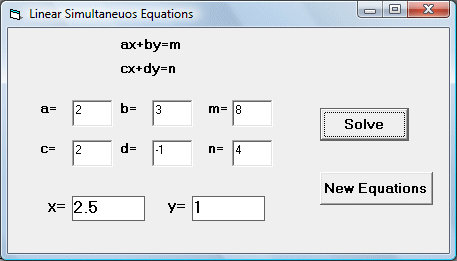# Simultaneous Linear Equations Solver

Simultaneous equations are equations that involves two or more unknown variables. There must be as many equations as the number of unknown variables in order for us to solve the problem. In this example, we shall only solve linear simultaneous equations. Linear simultaneous equations take the following forms:

ax+by=m

cx+dy=n

There are two ways to solve simultaneous equations, substitution or elimination. In this program, we use the substitution method. Reorganizing the equations derived the following formulas:

x = (b * n - d * m) / (b * c - a * d)
y = (a * n - c * m) / (a * d - b * c)

To limit the answers to two decimal places, we use the round function. The interface is shown in the Figure below:### The Code

Private Sub Solve_Click()
Dim a, b, c, d, m, n As Integer
Dim x, y As Double

a = Val(Txt_a.Text)
b = Val(Txt_b.Text)
m = Val(Txt_m.Text)
c = Val(Txt_c.Text)
d = Val(Txt_d.Text)
n = Val(Txt_n.Text)
x = (b * n - d * m) / (b * c - a * d)
y = (a * n - c * m) / (a * d - b * c)
Lbl_x.Caption = Round(x, 2)
Lbl_y.Caption = Round(y, 2)

End Sub

' To get new equations

Private Sub New_Click()
Txt_a.Text = ""
Txt_b.Text = ""
Txt_m.Text = ""
Txt_c.Text = ""
Txt_d.Text = ""
Txt_n.Text = ""
Lbl_x.Caption = ""
Lbl_y.Caption = ""

End Sub